# GMAT Math : DSQ: Calculating mode

## Example Questions

← Previous 1

### Example Question #1 : Dsq: Calculating Mode

What is the mode of a data set with ten data values?

1) The value 15 occurs four times in the data set.

2) The value 16 occurs three times in the data set.

BOTH statements TOGETHER are sufficient, but neither statement ALONE is sufficient.

Statement 2 ALONE is sufficient, but Statement 1 alone is not sufficient.

EACH statement ALONE is sufficient.

Statements 1 and 2 TOGETHER are not sufficient.

Statement 1 ALONE is sufficient, but Statement 2 alone is not sufficient.

BOTH statements TOGETHER are sufficient, but neither statement ALONE is sufficient.

Explanation:

If we are given only that 15 occurs four times in the data set, it is possible that another number can occur up to six times; similarly, if we are given only that 16 occurs three times, it is possible that another number can occur up to seven times. Either way, the mode - the most frequently occurring data value - cannot be determined.

However, if we know both facts, then no other data value can occur more than three times, so 15 must be the mode.

Therefore, the answer is that both statements are sufficient, but not one alone.

### Example Question #1 : Mode

Give the median of the data set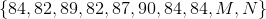,

whereandare integers.

1)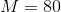2)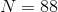BOTH statements TOGETHER are NOT sufficient to answer the question.

EITHER Statement 1 or Statement 2 ALONE is sufficient to answer the question.

BOTH statements TOGETHER are sufficient to answer the question, but NEITHER statement ALONE is sufficient to answer the question.

Statement 2 ALONE is sufficient to answer the question, but Statement 1 ALONE is not sufficient.

Statement 1 ALONE is sufficient to answer the question, but Statement 2 ALONE is not sufficient.

Statement 1 ALONE is sufficient to answer the question, but Statement 2 ALONE is not sufficient.

Explanation:

Supposebutis unknown. The above set, with known elements ordered, is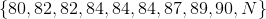The median is the arithmetic mean of the two middle elements, when the elements are ordered.

Regardless of the value of, the two middle elements must both be 84, making the median 84.

Now, supposebutis unknown. The above set, with known elements ordered, is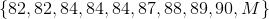The median cannot be determined with certainty. For example, if, as stated before, the median is 84. But if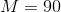, the middle elements are 84 and 87, making the median 85.5.

The answer is that Statement 1 alone is sufficent to answer the question, but Statement 2 alone is not.

### Example Question #1 : Dsq: Calculating Mode

The set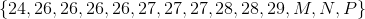is bimodal. What isequal to?

1)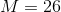2)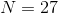Statement 2 ALONE is sufficient to answer the question, but Statement 1 ALONE is not sufficient to answer the question.
Statement 1 ALONE is sufficient to answer the question, but Statement 2 ALONE is not sufficient to answer the question.
BOTH statements TOGETHER are not sufficient to answer the question.
BOTH statements TOGETHER are sufficient to answer the question, but NEITHER statement ALONE is sufficient to answer the question.
EITHER statement ALONE is sufficient to answer the question.
Correct answer: Statement 1 ALONE is sufficient to answer the question, but Statement 2 ALONE is not sufficient to answer the question.
Explanation:

If we know that, then the set is known to have one 24, five 26's, three 27's, two 28's, and one 29. The only way the set can have two modes is forand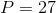; this makes 27 occur five times, just as frequently as 26.

If we know, however, the set  is known to have one 24, four 26's, four 27's, two 28's, and one 29. There are two ways for the set to have two modes (26 and 27): forand, or  for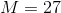and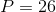The answer is that Statement 1 alone is sufficient to answer the question, but not Statement 2.

### Example Question #4 : Dsq: Calculating Mode

Consider this data set: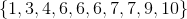Which of the following statements correctly compares the median and the mode?

The mode exceeds the median by 1.

The mode exceeds the median by 0.5.

The median exceeds the mode by 1.

The median exceeds the mode by 0.5.

The median and the mode are equal.

The median and the mode are equal.

Explanation:

The median of a data set with an even number of elements is the arithmetic mean of the two elements that fall in the middle when the elements are arranged in ascending order. These two elements are both 6, so 6 is the median.

The mode of a data set is the element that occurs most frequently. Since 6 appears thre times, 7 appears two times, and all other elements appear once each, the mode is 6.

Therefore, the median and the mode are equal.

### Example Question #2 : Mode

Consider the data set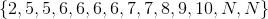What is the value of?

Statement 1: The data set is bimodal.

Statement 2: The mean of the data set is 6.5.

BOTH statements TOGETHER are insufficient to answer the question.

EITHER statement ALONE is sufficient to answer the question.

BOTH statements TOGETHER are sufficient to answer the question, but NEITHER statement ALONE is sufficient to answer the question.

Statement 2 ALONE is sufficient to answer the question, but Statement 1 ALONE is NOT sufficient to answer the question.

Statement 1 ALONE is sufficient to answer the question, but Statement 2 ALONE is NOT sufficient to answer the question.

Statement 2 ALONE is sufficient to answer the question, but Statement 1 ALONE is NOT sufficient to answer the question.

Explanation:

The data set has four 6's and no more than two of any other element - and there cannot be more than four of any other element regardless of the value of- so  6 must be one of the modes. For the set to be bimodal, there must be four of another element. Sinceoccurs twice, it must be set to a number known to occur exactly two other times. There are, however, two choices, 5 and 7, so Statement 1 is insufficient.

Statement 2 is sufficient, as seen below: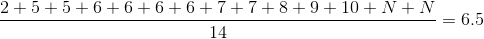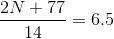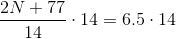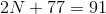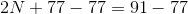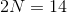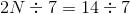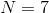### Example Question #6 : Dsq: Calculating Mode

Consider this data set: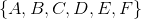with six unknown values.

How many modes does this data set have?

Statement 1: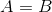.

Statement 2:  None of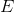, orare equal to each other.

BOTH statements TOGETHER are sufficient to answer the question, but NEITHER statement ALONE is sufficient to answer the question.

Statement 2 ALONE is sufficient to answer the question, but Statement 1 ALONE is NOT sufficient to answer the question.

Statement 1 ALONE is sufficient to answer the question, but Statement 2 ALONE is NOT sufficient to answer the question.

BOTH statements TOGETHER are insufficient to answer the question.

EITHER statement ALONE is sufficient to answer the question.

BOTH statements TOGETHER are sufficient to answer the question, but NEITHER statement ALONE is sufficient to answer the question.

Explanation:

If, as given in Statement 1, the set can have one mode (the other four numbers are different from each other and fromand), two modes (for example,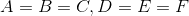) or three modes (for example,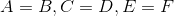) . Therefore, Statement 1 alone is not enough.

If none of, orare equal to each other, as given in Statement 2, the set can have one mode (for example,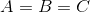, the other numbers are different), two modes (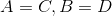andare different), or no modes (all six different numbers).

If both statements are true, however, there are two possibilities -, with the other four elements being different, or, with one number being the same and the other three different. Either way, the set is known to have one mode.

### Example Question #51 : Descriptive Statistics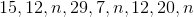What is the value of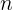in the list above?

(1)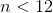(2) The mode of the numbers in the list is.

Statements (1) and (2) TOGETHER are not sufficient.

Statement (2) ALONE is sufficient, but Statement (1) ALONE is not sufficient.

Both statements TOGETHER are sufficient, but NEITHER statement ALONE is sufficient.

Each Statement ALONE is sufficient.

Statement (1) ALONE is sufficient, but Statement (2) ALONE is not sufficient.

Statement (2) ALONE is sufficient, but Statement (1) ALONE is not sufficient.

Explanation:The mode is the value that appears most often in a set of data. In our list the value that appears most often is n. Therefore n is the mode of the numbers in the list.

Only statement (2) is useful in finding the value of n as it states that the mode of the numbers in the list is 16.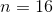### Example Question #1 : Dsq: Calculating Mode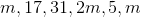What is the value ofin the list of numbers above?

(1)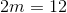.

(2) The mode of the numbers in the list is.

Statement (2) ALONE is sufficient, but Statement (1) ALONE is not sufficient.

Each Statement ALONE is sufficient.

Statements (1) and (2) TOGETHER are not sufficient.

Statement (1) ALONE is sufficient, but Statement (2) ALONE is not sufficient.

Both statements TOGETHER are sufficient, but NEITHER statement ALONE is sufficient.

Each Statement ALONE is sufficient.

Explanation:The mode is the value that appears most often in a set of numbers. In the list given, the value that appears the most is m. Therefore, m is the mode in the list of numbers given.

(1)Therefore,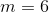.

Statement (1) is sufficient

(2) The mode of the numbers in the list is 6.

Therefore,.

Statement (2) is sufficient

Each Statement ALONE is SUFFICIENT

### Example Question #10 : Dsq: Calculating Mode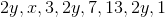What is the sum ofand?

(1) The mode of the numbers in the list is.

(2) The product ofandis.

Each Statement ALONE is sufficient.

Statements (1) and (2) TOGETHER are not sufficient.

Statement (1) ALONE is sufficient, but Statement (2) ALONE is not sufficient.

Statement (2) ALONE is sufficient, but Statement (1) ALONE is not sufficient.

Both statements TOGETHER are sufficient, but NEITHER statement ALONE is sufficient.

Both statements TOGETHER are sufficient, but NEITHER statement ALONE is sufficient.

Explanation:The mode is the value that appears most often in a set of data. In our list the value that appears most often is 2y. Therefore 2y is the mode of the numbers in the list.

(1) The mode of the numbers in the list is 20.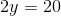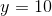We still don't know the value of x. Statement (1) ALONE is not sufficient.

(2) The product of x and y is 150.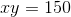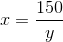Statement (2) ALONE is not sufficient.

Using both statements, we can write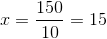Therefore,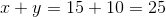Both statements TOGETHER are sufficient, but NEITHER statement ALONE is sufficient.

### Example Question #51 : Descriptive Statistics

Find the mode of the following set of numbers: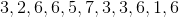The mode is the number that occurs most frequently. Therefore, our answer is.